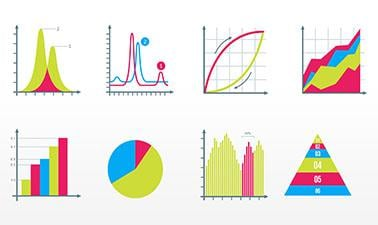## Friday, January 10, 2020

### Probability and Statistics in Data Science using PythonThe job of a data scientist is to glean knowledge from complex and noisy datasets. Reasoning about uncertainty is inherent in the analysis of noisy data. Probability and Statistics provide the mathematical foundation for such reasoning. In this course, part of the Data Science MicroMasters program, you will learn the foundations of probability and statistics. You will learn both the mathematical theory, and get a hands-on experience of applying this theory to actual data using Jupyter notebooks. Concepts covered included: random variables, dependence, correlation, regression, PCA, entropy and MDL.